# Texas Go Math Grade 1 Lesson 15.2 Answer Key Attributes of Three-Dimensional Solids

Refer to our Texas Go Math Grade 1 Answer Key Pdf to score good marks in the exams. Test yourself by practicing the problems from Texas Go Math Grade 1 Lesson 15.2 Answer Key Attributes of Three-Dimensional Solids.

## Texas Go Math Grade 1 Lesson 15.2 Answer Key Attributes of Three-Dimensional Solids

Explore

Draw to sort the three-dimensional solids.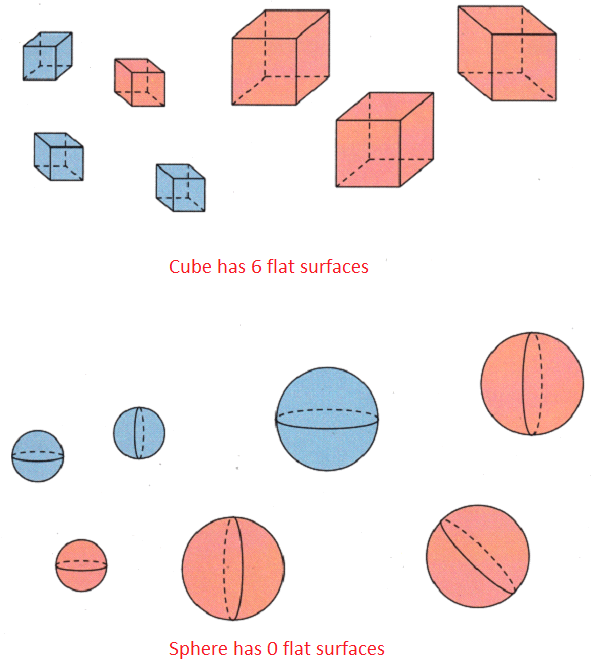FOR THE TEACHER • Have children sort the three-dimensional solids into two groups. Have them draw around each group to show how they sorted.

Math Talk
Mathematical Processes

Explain how you sorted the solids.
Answer: Cube has 6 flat surfaces and Sphere has 0 flat surfaces.

Model and Draw

Look at the surfaces on these three-dimensional solids. Some are flat. Some are curved.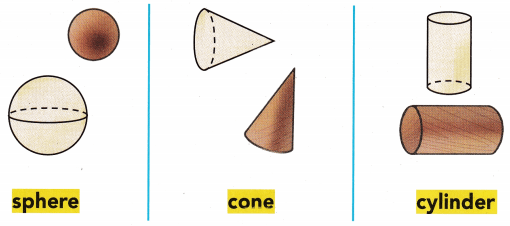Share and Show

Use spheres, cones, and cylinders. Sort the solids into two groups. Name and draw the solids.

Question 1.
only a curved surface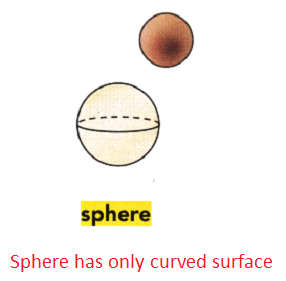Explanation:
A sphere has only a curved surface with no flat and curved surfaces.

Question 2.
both flat and curved surfaces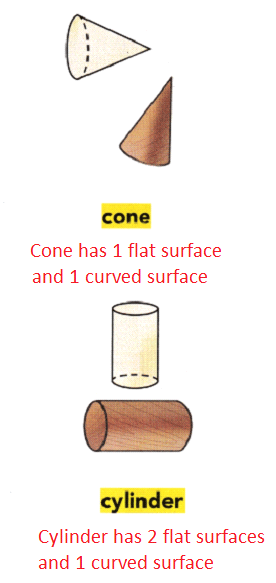Explanation:
A cone has 1 flat surface and 1 curved surface,
A cylinder has 2 flat surfaces and 1 curved surface.

Problem Solving

Use three-dimensional solids. Write the number of flat surfaces for each shape. Exercise 3-8 can help you write the shape names

Question 3.
A cylinder has _________ flat surfaces.
Answer: A cylinder has 2 flat surfaces.

Question 4.
A cone has ___________ flat surface.
Answer: A cone has 1 flat surface.

Question 5.
A sphere has _________ flat surfaces.
Answer: A sphere has no flat surfaces.

H.O.T. Write to name each shape.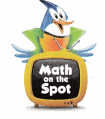Question 6.Question 7.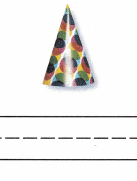Question 8.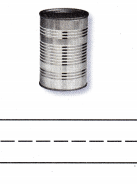Question 9.
H.O.T. Multi-Step Kelly drew objects that have both flat and curved surfaces. Circle the objects she drew.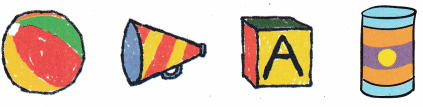Question 10.
Lorna wins a toy that has no flat surfaces. Which solid ¡s the shape of the toy she wins?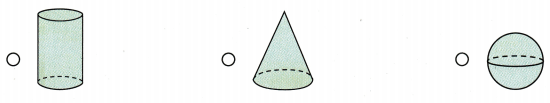Answer: A sphere has no flat surface, Lorna wins a sphere shaped toy.

Question 11.
Analyze Gerri has a can that is shaped like a cylinder. How many flat surfaces does the can have?
(A) 2
(B) 4
(C) 1
A cylinder has 2 flat surfaces, Gerri has a can that is shaped like a cylinder with 2 flat surfaces.

Question 12.
Which solid has only 1 flat surface?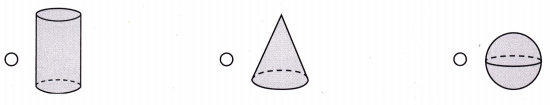Answer: A cone has only 1 flat surface.

Question 13.
Texas Test Prep Which solid has 2 flat surfaces?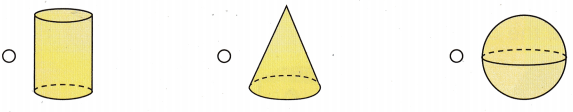Answer: A cylinder has 2 flat surfaces.

TAKE HOME ACTIVITY • Ask your child to name real objects shaped like a sphere, a cone, and a cylinder.

### Texas Go Math Grade 1 Lesson 15.2 Homework and Practice Answer KeyDraw each solid.

Question 1.
cone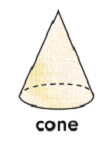Question 2.
cylinderQuestion 3.
sphereProblem Solving

Write the name of each solid shape.

Question 4.
Ahas 0 flat surfaces.

Question 5.
Ahas 1 flat surface.
Answer: A cone has 1 flat surface.

Question 6.
Ahas 2 flat surfaces.
Answer: A cylinder has 2 flat surfaces.

Lesson Check

Question 7.
Emma plays a music toy with a curved surface and 2 flat surfaces. Which solid is the shape of the toy she plays?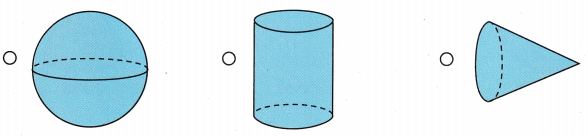Answer: A cylinder has 1 curved surface and 2 flat surfaces, Emma plays with a musical toy which is in cylindrical shape.

Question 8.
Jason has a toy shaped like a sphere. How many flat surfaces does the toy have?
(A) 0
(B) 1
(C) 2
Answer: A sphere has 0 flat surface, Jason toy has 0 flat surface

Question 9.
Bailey used blocks to build a tower. She used blocks with 2 flat surfaces. Which block did she use?Answer: A cylinder has 2 flat surfaces and 1 curved surface, Bailey uses cylindrical blocks to build a tower.

Question 10.
Multi-Step Which solid has a flat surface and a curved surface?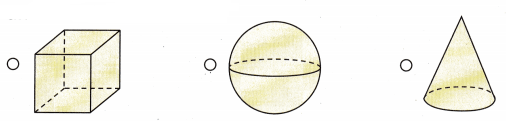Answer: A cone has 1 flat surface and 1 curved surface.

Scroll to Top